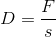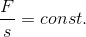## Hooke’s law

Describes the linear correlation according to eq. 1 between the elastic deformation of a body and the force required for this deformation.

Eq. 1:D = spring constant [N/m]
F = deflection force [N]
S = deflection[m]

The standard example for the applicability of Hooke’s law is the elongation of compression springs. If different weights are applied, the compression spring shows different elongations compared to the initial state.

The deflection of the weight force is proportional within a certain range which is referred to as proportionality range. This is illustrated in eq. 2:

Eq. 2:A spring with a spring constant of 1 N/m is consequently elongated by 1 m if a force of 1 Newton is applied; in case of a spring constant of 50, 50 N are required for the same deflection. Thus, the spring is the tighter the larger the value for D.